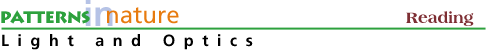The Photoelectric Effect

The focus in this course is on the interaction of electromagnetic waves (photons) with electrons to understand the creation and absorption of light. Electromagnetic waves have wavelengths that range from 1 nanometer (10-9 meter) for x-rays to 1 kilometer (103 meters) for radiowaves. Wavelengths of visible light are in the range of 400 to 700 nanometers (nm). In this appendix we consider the formulation of photon wavelength and energy as well as the absorption of a photon by an electron.

Moving waves can be described by their frequency, or number of waves per second, as well as by their wavelengths. If a train of waves is moving past a stationery observer at a frequency of f complete cycles, or waves per second, and if each of these has a wavelength (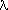) of centimeters (cm) per cycle, then the wave must be moving at a speed of v cm per second (s). The velocity (v), wavelength () and frequency (f) are related by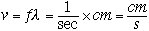(1)

All electromagnetic radiation, radio waves through x-rays, moves in vacuum at a universal speed. This is the speed of light, c=30,000,000,000 centimeters per second (usually written in powers of ten, c=3x1010 cm/sec). The constant value of the speed of light in vacuum goes against our intuition: we would expect that high energy (short wavelength) radiation would move faster than low energy (long wavelength) radiation. We can consider light as a stream of minute packets of energy, photons, which create a pulsating electromagnetic disturbance. A single photon differs from another photon only by its energy. In empty space (vacuum) all photons travel with the same speed or velocity. Photons are slowed down when they pass through different media such as water, glass or even air. This slowing down accounts for the refraction or bending of light by optical lenses. The energy of the photon is not changed, but the wavelength is. Different energy optical photons are slowed by different amounts in glass or water; this leads to the dispersion of light and the appearance of rainbows.

Because the speed of light in vacuum is constant, if we know either the frequency of the wavelength of electromagnetic radiation we can calculate the other quantity from equation 1. The frequency of the electromagnetic vibration we call light is given in units of "hertz" (abbreviated Hz and named after Henrich Hertz, 1857-1894, a famous German investigator of electromagnetism). One hertz is one vibration per second; the range of the pure spectrum perceived by the eye extends from about 4.3x1014 Hz in the red to about 7.5x1014 in the violet.

This aspect of the discussion suggests that light and other electromagnetic radiation are waves. It was very disturbing, therefore, when phenomena were discovered (around 1900) that clearly indicated that light was made up of particles called photons. One such phenomenon involved the photoelectric effect. It was known that if one shines a beam of light on a clean surface of a metal, electrons will be ejected from the metal. The light has to exceed a certain energy, to remove electrons from the metal surface. If the light has more than the minimum energy required, then the extra energy will be given to the ejected electrons as kinetic energy of motion.

Light of a single wavelength behaved as if it consisted of separate particles, photons, all with the same energy, with each ejected electron being the result of a collision between one photon and one electron in the metal. Greater intensity of light meant only that more photons were hitting the metal per second and more electrons were being ejected, not that there was more energy per photon. The energy of the outgoing electrons depended on the frequency of light used.

The energy (E) of the incoming photons is directly proportional to the light frequency which can be written as,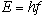(2)

in which h is a constant.

Max Planck first proposed this relationship between energy and frequency in 1900 as part of his study of the way in which heated solids emit radiation. The constant h is called Planck's constant in his honor and has a value, h=4.136x10-15 eV-sec.

For photons, the property most readily measured is their energy. The different colors of light for example, are thought of as representing photons of different energy. The link between the particle theory and the wave theory lies in Planck's fundamental postulate of quantum theory given by equation (2).

There is an inverse relation between the energy E, of the photon and the wavelength,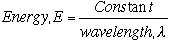(3)

The energy E in equation 3 can be expressed in many units. In the analysis of art and in the description of light, the most convenient unit of energy to use is the electron volt, abbreviated eV.

In terms of wavelength,, in nanometers (nm), and energy (E), in electronvolts (ev), equation 3 can be expressed for light travelling in a vacuum as: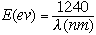(4)

as show in Fig. 1.

The constants and numerical relations found in equations 3 and 4 are found from Planck's equation (Eqn. 2) by writing the relationship for frequency (f) in Eqn. 1 as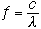(5)

so that Planck's equation becomes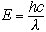(6)

The value of Planck's constant, h, is 4.136x10-15 eV-sec and the velocity of light, c, is 3x108 m/sec or 3x1017 nm/sec so that hc=12.4x102 or 1240 eV-nm.

Visible light is composed of photons in the energy range of around 2 to 3 eV. Orange light with a wave length of 620 nanometers is composed of photons with energy of 2 eV. It is the energy range of 2 to 3 eV which triggers the photo receptors in the eye. Lower energies (longer wavelengths) are not detected by the human eye but can be detected by special semiconductor infared sensors. Higher energies (shorter wavelengths) such as x-rays damage human tissue and are detected by x-ray sensitive photographic film or again by special semiconductor devices.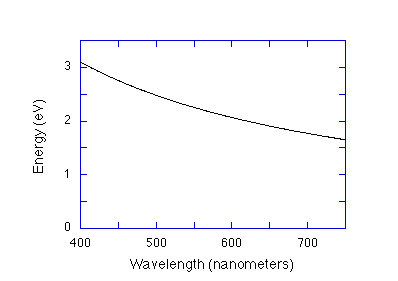Figure 1. A curve that shows the relationship, E(ev)=1240/(nm) given in Eqn. 4 for the energy in eV versus photon wavelength in nanometers for the visible region of the electromagnetic spectrum.